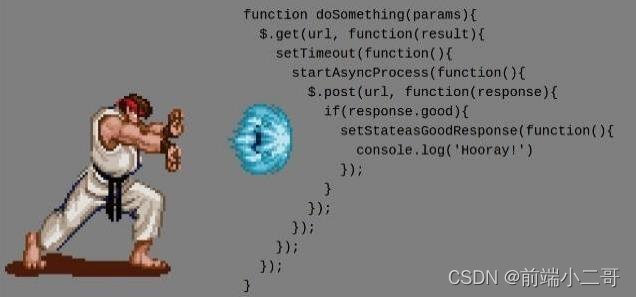> 文档中心 > 手写Promise--第一篇

# 前言### 文章の目录

• 前言
• 手写Promise前必读
• 🥇 实现Promise的基本功能
• 🥇 then()方法的实现
• 写在最后

# 手写Promise前必读

📣注意：在Promise的状态发生改变后就会发生状态凝固，即状态不能再次改变；

# 🥇 实现Promise的基本功能``class Promise {  constructor(executor) {    //调用执行器函数    executor(resolve, reject)  }}````class Promise {  constructor(executor) {    this.state = 'pending'//Promise初始状态    this.value = undefined//执行成功的结果    this.reason = undefine//执行失败的结果    //调用执行器函数    executor(resolve, reject)  }}``

``class Promise {  constructor(executor) {    this.state = 'pending' //Promise初始状态    this.value = undefined //执行成功的结果    this.reason = undefine //执行失败的结果    // 定义resolve函数    const resolve = (value) => {      if (this.state === 'pending') { this.state = 'fulfiled' this.value = value      }    }    //定义reject函数    const reject = (reason) => {      if (this.state === 'pending') { this.state = 'rejected' this.reason = reason      }      //调用执行器函数      executor(resolve, reject)    }  }}``

``//捕获Promise状态发生错误的第三种方式     try {      //调用执行器函数 executor(resolve, reject)     } catch (err) {      reject(err)     }``

# 🥇 then()方法的实现

``class Promise {  constructor(executor) {    this.state = 'pending' //Promise初始状态    this.value = undefined //执行成功的结果    this.reason = undefine //执行失败的结果    // 定义resolve函数    const resolve = (value) => {      if (this.state === 'pending') { this.state = 'fulfiled' this.value = value      }    }    //定义reject函数    const reject = (reason) => {      if (this.state === 'pending') { this.state = 'rejected' this.reason = reason      }      //调用执行器函数      executor(resolve, reject)    }  }  then(onResolved, onRejected) {    //Promise状态为resolved时    if (this.state === 'resolved') {      onResolved(this.value)    }    //Promise状态为rejected时    if (this.state === 'rejected') {      onRejected(this.reason)    }  }}``

``var myP = new Promise(function(resolve, reject){    console.log('执行')    setTimeout(function(){ reject(3)    }, 1000)});myP.then(function(res){    console.log(res)},function(err){    console.log(err)});````then(onResolved, onRejected) {    //Promise状态为resolved时    if (this.state === 'resolved') {      onResolved(this.value)    }    //Promise状态为rejected时    if (this.state === 'rejected') {      onRejected(this.reason)    }    // 如果状态为pending，将回调函数存如数组    if (this.state === 'pending') {      //将执行成功的回调函数存入数组      this.resolvedCallback.push(() => { onResolved(this.value)      })    }    //将执行失败的回调函数存入数组    this.rejectedCallback.push(() => {      onRejected(this.reason)    })  }``

``class Promise {  constructor(executor) {    this.state = 'pending' //Promise初始状态    this.value = undefined //执行成功的结果    this.reason = undefine //执行失败的结果    //定义数组用来存放回调函数    this.resolvedCallback = []    this.rejectedCallback = []    // 定义resolve函数    const resolve = (value) => {      if (this.state === 'pending') { this.state = 'fulfiled' this.value = value //  改变完状态执行数组中的回调函数 this.resolvedCallback.forEach((item) => item())      }    }    //定义reject函数    const reject = (reason) => {      if (this.state === 'pending') { this.state = 'rejected' this.reason = reason // 改变完状态执行数组中的回调函数 this.rejectedCallback.forEach((item) => item())      }      //调用执行器函数      executor(resolve, reject)    }  }  then(onResolved, onRejected) {    //Promise状态为resolved时    if (this.state === 'resolved') {      onResolved(this.value)    }    //Promise状态为rejected时    if (this.state === 'rejected') {      onRejected(this.reason)    }    // 如果状态为pending，将回调函数存如数组    if (this.state === 'pending') {      //将执行成功的回调函数存入数组      this.resolvedCallback.push(() => { onResolved(this.value)      })    }    //将执行失败的回调函数存入数组    this.rejectedCallback.push(() => {      onRejected(this.reason)    })  }}``

# 写在最后

🥂(❁´◡`❁)您的点赞👍➕评论📝➕收藏⭐是作者创作的最大动力🤞